Function Repository Resource:

# CellularAutomatonNumber

Convert an explicit list of rules specifying a cellular automaton into the corresponding rule number

Contributed by: Wolfram Physics Project Team
 ResourceFunction["CellularAutomatonNumber"][rules] converts a cellular automaton specified by rules into an equivalent rule number.

## Details and Options

The result is returned as a List {n,k,r}, where n is the rule number, k is the number of colors and r is the range.

## Examples

### Basic Examples (2)

Generate the elementary rule number for a cellular automaton from a List of rules:

 In:=Out=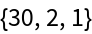Show that the two specifications are equivalent:

 In:=Out=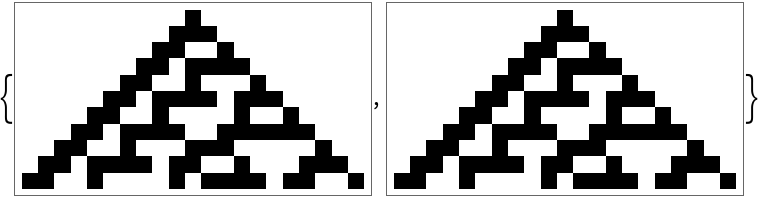### Scope (1)

Generate rule numbers for cellular automata with different colors and ranges:

 In:=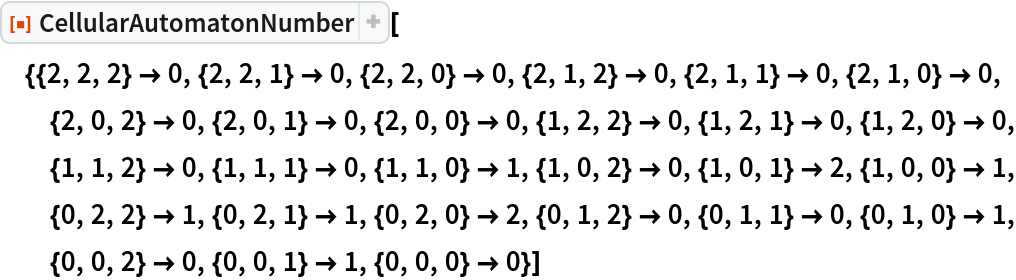Out=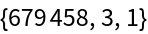In:=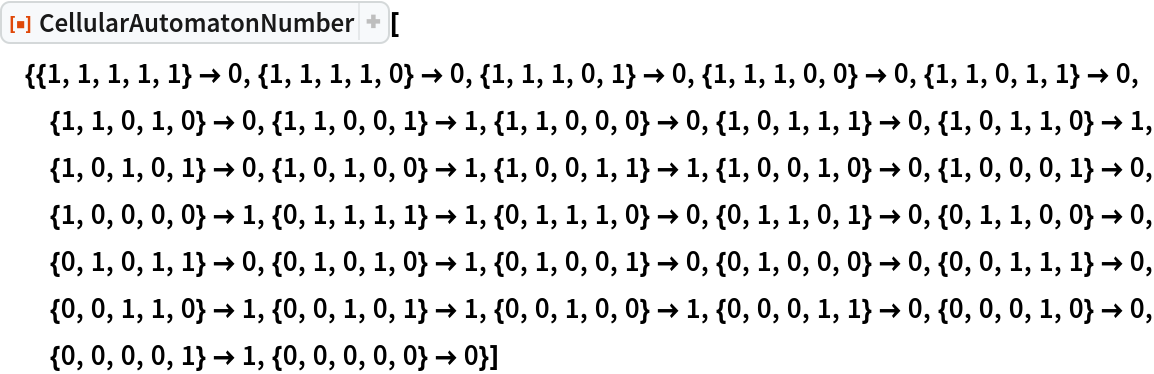Out=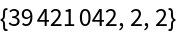## Version History

• 1.0.0 – 12 August 2020